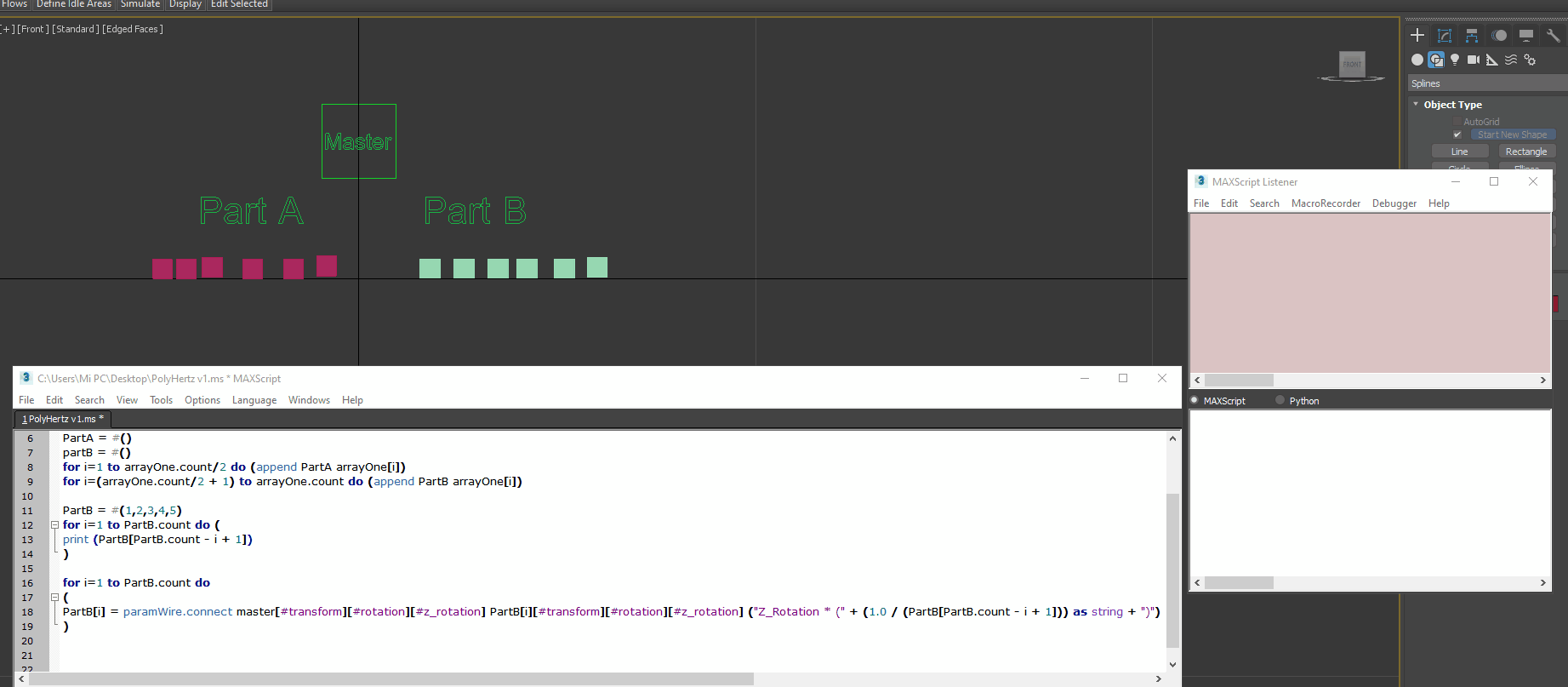# 3DSMAX Maxscript cut array in 1/2?

Offline / Send Message
polycounter lvl 5
I need to divide 1 array of objects into 2 and each part can create a new array with its own name.

## Replies

• Offline / Send Message
ngon master
You mean like this?

arrayOne = #(1,5,7,23,9,5,65)
PartA = #()
partB = #()
for i=1 to arrayOne.count/2 do (append PartA arrayOne[i])
for i=(arrayOne.count/2 + 1) to arrayOne.count do (append PartB arrayOne[i])
• Offline / Send Message
polycounter lvl 5
Thank you very much, that's exactly what I was needing
• Offline / Send Message
polycounter lvl 5
PolyHertz said:
You mean like this?

arrayOne = #(1,5,7,23,9,5,65)
PartA = #()
partB = #()
for i=1 to arrayOne.count/2 do (append PartA arrayOne[i])
for i=(arrayOne.count/2 + 1) to arrayOne.count do (append PartB arrayOne[i])
A question that is related to the previous one.
Now that I have 2 object arrays the second array starts connecting the values but from 0.0 to 1.0 and I would need the opposite starting with a value of 1.0 and ending with a value of 0.0
I'm using this way to do it and it's not the right way.
```</code>("Z_Rotation * (" + ((1.0 / PartB.count )* i) as string + ")")</pre><br></div><div><br><img alt="" src="https://us.v-cdn.net/5021068/uploads/editor/yf/z282uv9oyjm4.gif" title="Image: https://us.v-cdn.net/5021068/uploads/editor/yf/z282uv9oyjm4.gif"><br><div><pre class="CodeBlock"><code>master = \$master
slave = \$slave???
select slave
arrayOne = selection as array

PartA = #()
partB = #()
for i=1 to arrayOne.count/2 do (append PartA arrayOne[i])
for i=(arrayOne.count/2 + 1) to arrayOne.count do (append PartB arrayOne[i])

for i=1 to PartB.count do
(
PartB[i] = paramWire.connect master[#transform][#rotation][#z_rotation] PartB[i][#transform][#rotation][#z_rotation] ("Z_Rotation * (" + ((1.0 / PartB.count )* i) as string + ")")
)```

• Offline / Send Message
ngon master
You can count backwards by subtracting the current loop number from the highest array index:

PartB = #(1,2,3,4,5)
for i=1 to PartB.count do (
print (PartB[PartB.count - i + 1])
)

So in your example it would probably look something like this: EDIT, see below!
• Offline / Send Message
polycounter lvl 5
PolyHertz said:
You can count backwards by subtracting the current loop number from the highest array index:

PartB = #(1,2,3,4,5)
for i=1 to PartB.count do (
print (PartB[PartB.count - i + 1])
)

So in your example it would probably look something like this:

`PartB[i] = paramWire.connect master[#transform][#rotation][#z_rotation] PartB[i][#transform][#rotation][#z_rotation] ("Z_Rotation * (" + (1.0 / (PartB[PartB.count - i + 1])) as string + ")")`

This part of the code works perfectly
`PartB = #(1,2,3,4,5)for i=1 to PartB.count do (    print (PartB[PartB.count - i + 1]))`
When connecting the wire parameter, an error occurs and it cannot connect to the elements.The sample 3dsmax scene to test.
`PartB[i] = paramWire.connect master[#transform][#rotation][#z_rotation] PartB[i][#transform][#rotation][#z_rotation] ("Z_Rotation * (" + ((1.0 / PartB.count) * (PartB.count - i + 1)) as string + ")")`
`PartB[i] = paramWire.connect master[#transform][#rotation][#z_rotation] PartB[i][#transform][#rotation][#z_rotation] ("Z_Rotation * (" + ((1.0 / PartB.count) * (PartB.count - i + 1)) as string + ")")`